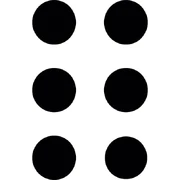## FANDOM

10,407 Pages

6 (six) is a positive integer following 5 and preceding 7. Its ordinal form is written "sixth" or "6th".

## PropertiesVisualization of 6 6 is an even number, the third triangular number, the third factorial number, and 3 or 4 primorial.

6 is equal to the sum of its proper divisors: 1 + 2 + 3 = 6. This makes it a perfect number, and in fact the smallest number with that property, with the next being 28.

6 is equal to the sum of its unitary proper divisors, that is to say divisors d such that d and n/d are relatively prime. These are called unitary perfect numbers. It is the smallest number with this property.

It is the only semiprime number to not also be a deficient number.

It is the smallest number N such that all multiples of N are abundant. All perfect or abundant numbers have this property.

In Czech language, 6 is called "půltucet", meaning "half-twelve".

## In googology

Some googologisms based on 6 are sextoogol, superhex and

hexalogue. It is not commonly found in googology.

Six is the basis of Robert Munafo's idea of classes. 6 is the boundary between class 0 and class 1 numbers, 106 is the boundary between class 1 and class 2 numbers, 10106 is the boundary between class 2 and class 3 numbers, and so on.

The Misalian seximal system uses base six, which is also called senary or heximal.

In Greek-based number naming systems, 6 is associated with prefix hexa-, and with prefix sexti- in Latin systems.

6 is the last digit of mega.